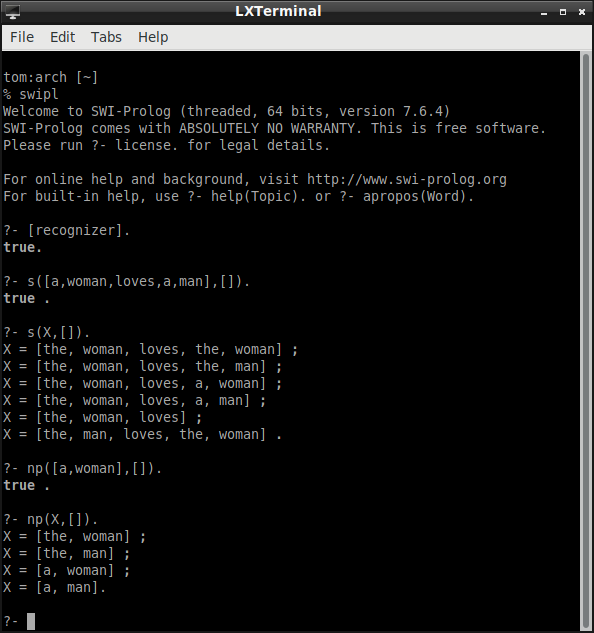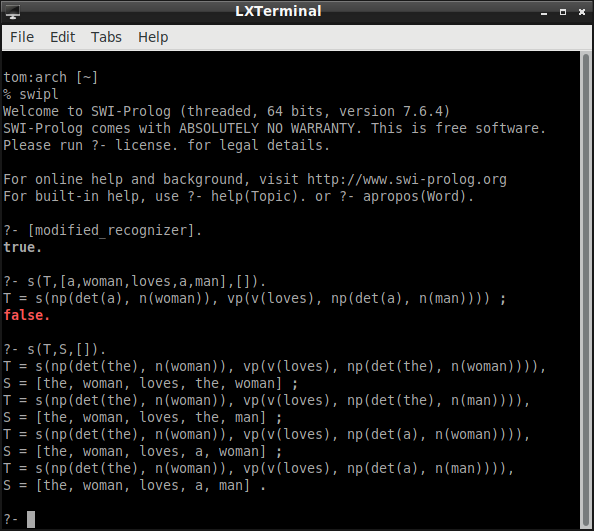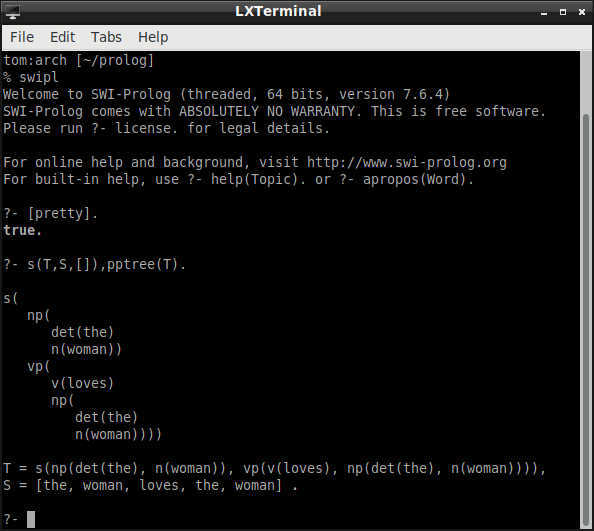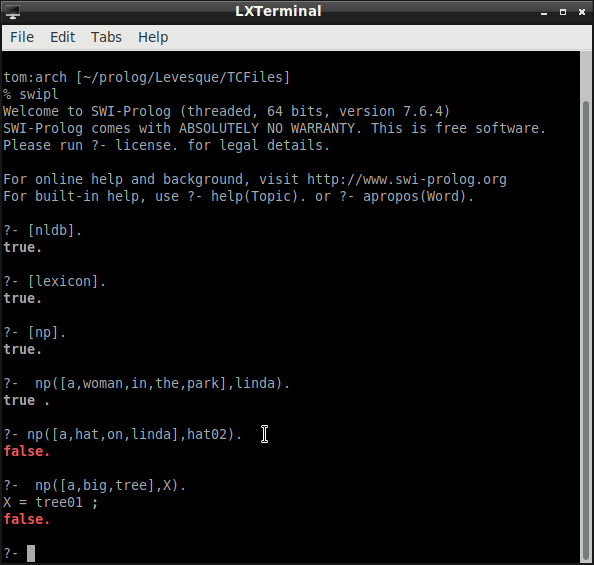# Philosophy, Computing, and Artificial Intelligence

PHI 319. Understanding Natural Language.

Thinking as Computation
Chapter 8 (153-176)

## Natural Language Processing

Human beings understand language. How do they do it?

It would seem that human beings, or any intelligent agent who understands natural language, must process the words they see and hear in terms of a lexicon and a grammar.

### A Lexicon and A Grammar

A language is defined by a lexicon and a grammar. Here is a simple example:

s -> np vp
np -> det n
vp -> v np
vp -> v
det -> a
det -> the
n -> woman
n -> man
v -> loves

The symbols s, np, vp, det, n, and v stand for grammatical categories:

s:      sentence
np:   noun phrase
vp:   verb phrase
det:  determiner

The symbols s, np, and vp are non-terminal grammatical categories. The symbols det, n, and v are terminal grammatical categories. The symbols a, the, woman, man, and loves are the lexical items in the terminal grammatical categories.

### A Sentence of the Language

Consider the string of words a woman loves a man. Is this string grammatical? That is to say, is it a member of the language given by the example lexicon and grammar?

The following parse tree shows that the string is sentence of the language:

```                              s
|
|
/                        \
np                              vp
|                                |
|                                |
/       \                       /        \
det            n                 v               np
/         \
|             |                |
a           woman       loves      det           n
|            |
a           man
```

## A Recognizer Written in Prolog

A "recognizer" is a program that recognizes whether a given string is in the language. Here is a recognizer (written in Prolog) for the language given by the lexicon and grammar:

s(X,Z) :- np(X,Y), vp(Y,Z).
np(X,Z) :- det(X,Y), n(Y,Z).
vp(X,Z) :- v(X,Y), np(Y,Z).
vp(X,Z) :- v(X,Z).

det([the|W],W).
det([a|W],W).

n([woman|W],W).
n([man|W],W).

v([loves|W],W).

The recognizer works in terms of what are called "difference lists."

A difference list represents a list in terms of a pair of lists. The list represented is the "difference" between the two lists. So, for example,

[a,woman,loves,a,man]

is the "difference" between the lists

[a,woman,loves,a,man] and [].

In the recognizer, the np predicate looks for a noun phrase at the beginning of the first list. If the np predicate finds a noun phrase ([a,woman]), it passes what remains in the list ([loves,a,man]) to the vp predicate. The vp predicate looks for a verb phrase at the beginning of the list it is given. If the vp predicate finds a verb phrase, and the difference between the list it is given and the verb phrase it finds is the second list (in this case []), then the query is successful.

An example helps show how it works. The query

s([a,woman,loves,a,man],[]).

asks whether the difference between the list

[a,woman,loves,a,man]

and the list

[]

is a list that contains a sentence of the language.

By unifying X with [a,woman,loves,a,man] and unifying Z with [], the query matches the head of the first rule. The query list thus becomes

np([a,woman,loves,a,man],Y), vp(Y,[]).

Prolog renames variables because sometimes unification is not possible otherwise. Consider the KB

f (a, X).

Suppose the query is

?- f( X, b)

This query is a logical consequence of the KB

∀x f(a, x)
---------- ∀E
f (a, b)
---------- ∃I
∃x f (x, b)

Unification, however, is not possible unless the variables are first standardized apart.
The variable Y in this derived query list clashes with the variable Y in the rules. So, to prevent mistakes in the computation, the variable Y in the derived query list is replaced

np([a,woman,loves,a,man],Y1), vp(Y1,[]).

By unifying X with [a,woman,loves,a,man] and unifying Z with Y1, the first query in this derived query list matches the head of the second rule. So the derived query list becomes

det([a,woman,loves,a,man],Y), n(Y,Y1), vp(Y1,[]).

By unifying Y and W with [woman,loves,a,man], the first item on this derived query list matches the second fact about determiners. (The vertical line ( | ) in the fact separates the head from the tail in the list. So, in the fact, the head is the determiner a.) Now the derived query is

n([woman,loves,a,man],Y1), vp(Y1,[]).

By unifying W and Y1 with [loves,a,man], the first item on the derived query list matches the first fact about nouns. Now the derived query is

vp([loves,a,man],[]).

This completes the computation of the noun phrase a woman. The computation of the verb phrase proceeds similarly, and it should be clear that the computation will eventually succeed.

Here is a screen shot of the recognizer at work:It is easy to modify the recognizer so that it displays the parse tree associated with the sentence:

s(s(NP,VP),X,Z) :- np(NP,X,Y), vp(VP,Y,Z).
np(np(DET,N),X,Z) :- det(DET,X,Y), n(N,Y,Z).
vp(vp(V,NP),X,Z) :- v(V,X,Y), np(NP,Y,Z).
vp(vp(V),X,Z) :- v(V,X,Z).

det(det(the),[the|W],W).
det(det(a),[a|W],W).

n(n(woman),[woman|W],W).
n(n(man),[man|W],W).

v(v(loves),[loves|W],W).

Again, an example makes it clearer how the program works. Suppose that the query is

s(T,[a,woman,loves,a,man],[]).

By unifying T with s(NP,VP), X with [a,woman,loves,a,man], and Z with [], the query matches the head of the first rule. The derived query list is

np(NP,[a,woman,loves,a,man],Y), vp(VP,Y,[]).

The variable Y in the derived query list clashes with the variable Y in the KB, so it is replaced in the derived query list

np(NP,[a,woman,loves,a,man],Y1), vp(VP,Y1,[]).

By unifying NP with np(DET,N), X with [a,woman,loves,a,man], and Y1 with Z, the first item on the derived query list matches the head of the second rule. The derived query list becomes

det(DET,[a,woman,loves,a,man],Y), n(N,Y,Y1), vp(VP,Y1,[]).

By unifying DET with det(a) and W and Y with [woman,loves,a,man], the first item on the derived query list matches the second fact about determiners. The derived query becomes

n(N,[woman,loves,a,man],Y1), vp(VP,Y1,[]).

Notice that in the computation so far, T = s(NP,VP), NP = np(DET,N), and DET = det(a). So, at this point in the computation, T = s(np(det(a),N).

It is easier to appreciate the (partially) computed value of T if it is presented in the form of a parse tree. Once the paretheses are replaced with branches, T is the following tree

```                           s
/
np
/    \
det     N
|
a
```

The rest of the computation works similarly, and it is clear that eventually the computation will succeed and return the computed value for T.

Here is a screen shot of the modified recognizer at work:I took this printer, with slight modification, from a course on Prolog given at the 16th European Summer School in Logic, Language, and Information. To make the parse trees easier to read, we can add a "pretty printer"## Models of the World

Understanding natural language requires more than the ability to recognize sentences of the language as grammatical. This is the ability to recognize that a string is grammatical. Understanding requires knowledge of the conditions under which sentences are true or false. For this, we need a model of the world against which sentences are evaluated as true or false.

A model shows what is true in the world.Here is an example (nldb.pl), written in Prolog, that Levesque provides:

```person(john). person(george). person(mary). person(linda).
park(kew_beach). park(queens_park).
tree(tree01). tree(tree02).  tree(tree03).
hat(hat01).   hat(hat02).  hat(hat03).  hat(hat04).

sex(john,male).    sex(george,male).
sex(mary,female).  sex(linda,female).

color(hat01,red).   color(hat02,blue).
color(hat03,red).   color(hat04,blue).

in(john,kew_beach).     in(george,kew_beach).
in(linda,queens_park).  in(mary,queens_park).
in(tree01,queens_park). in(tree02,queens_park).
in(tree03,kew_beach).

beside(mary,linda). beside(linda,mary).

on(hat01,john). on(hat02,mary). on(hat03,linda). on(hat04,george).

size(john,small).    size(george,big).
size(mary,small).    size(linda,small).
size(hat01,small).   size(hat02,small).
size(hat03,big).     size(hat04,big).
size(tree01,big).    size(tree02,small).  size(tree03,small).
```

This model is straightforward. There are four persons. Their names are "john," "george," "mary," and "linda." There are two parks. There are four trees, and so on. In addition to the objects in the model (the people, parks, and so on), the model specifies certain basic truths about the objects.

The model is a representation of things in the world. The connection of words to things in the world is given in terms of the "extensions" of the words to things in the world. The rules that define these extensions are in the lexicon. In this way, a lexicon is built in terms of a model.

Here is an example (lexicon.pl) that Levesque provides:

```article(a).  article(the).

common_noun(park,X) :- park(X).
common_noun(tree,X) :- tree(X).
common_noun(hat,X) :- hat(X).
common_noun(man,X) :- person(X), sex(X,male).
common_noun(woman,X) :- person(X), sex(X,female).

preposition(on,X,Y) :- on(X,Y).
preposition(in,X,Y) :- in(X,Y).
preposition(beside,X,Y) :- beside(X,Y).

% The preposition 'with' is flexible in how it is used.
preposition(with,X,Y) :- on(Y,X).        % Y can be on X
preposition(with,X,Y) :- in(Y,X).        % Y can be in X
preposition(with,X,Y) :- beside(Y,X).    % Y can be beside X

% Any word that is not in one of the four categories above.
proper_noun(X,X) :- \+ article(X), \+ adjective(X,_), \+ common_noun(X,_), \+ preposition(X,_,_).

```

Consider the first line in lexicon.pl.

It says that the words a and the belong to the grammatical category of article.

Consider the first rule for the category of common noun.

The first rule says that the word park belongs to the grammatical category of common noun and that something is in the extension of the word park if this thing is a park.

To understand this, return to what the model says about the parks in the world. According to the model, a place in the world whose name is "kew_beach" is a park. According to the model, the other park in the world is a place whose name is "queens_park." Together, these two parks (kew_beach and queens_park) constitute the extension of the common noun park.

Consider the third rule for the category of common noun.

This rule says that the word man belongs to the grammatical category of common noun and that something is in the extension of the word man if this thing is a person and is male. According to the model, the things whose names are "john" and "george" are the things in the world who are persons and male. Together, they constitute the extension of the common noun man.

It is possible to write a program that determines if an object is in the extension of a noun phrase.

Given the model and lexicon, the query

np([a,woman,in,a,park],linda)

succeeds because "linda" is the name of something in the extension of the noun phrase "a woman in a park." According to the model, the following are true: Linda is in Queens Park (in(linda,queens_park)) and Queens Park is a park (park(queens_park)).

On the other hand, the query

np([a,hat,on,linda],hat02)

fails because the hat whose name is "hat02" is not in the extension of the noun phrase "a hat on Linda." "hat02" is the name of a hat (hat(hat02)) that is on Mary (on(hat02,mary)).

Here is the Prolog program (np.pl) Levesque provides:

```np([Name],X) :- proper_noun(Name,X).
np([Art|Rest],X) :- article(Art), np2(Rest,X).

np2([Noun|Rest],X) :- common_noun(Noun,X), mods(Rest,X).

mods([],_).
mods(Words,X) :-
append(Start,End,Words),   % Break the words into two pieces.
pp(Start,X),                           % The first part is a PP.
mods(End,X).                       % The last part is a Mods again.

pp([Prep|Rest],X) :- preposition(Prep,X,Y), np(Rest,Y).

```

Consider the noun phrase "a big tree." The corresponding parse tree is

```                          NP
|
/              \
article            NP2
|                    |
|              /            \
|           |                      |
|           |                  /        \
|           |    common_noun  Mods
|           |                 |
a          big               tree

```

Here is in the computation to determine if tree01 is in the extension of "a big tree":

```
np([a,big,tree],tree01)                    query

|                                np([Art|Rest],X) :- article(Art), np2(Rest,X)
|                                Art = a, Rest = [big,tree], X = tree01

article(a), np2([big,tree],tree01)

|                                article(a) succeeds; matches fact in lexicon
|

np2([big,tree],tree01)

|                                Adj = big, Rest = tree, X = tree01

|                                X = tree01

size(tree01,big), np2(tree,tree01)

|                                size(tree01,big) succeeds; matches fact in model
|

np2(tree,tree01)

|                                np2([Noun|Rest],X) :- common_noun(Noun,X), mods(Rest,X)
|                                Noun = tree, Rest = [], X = tree01

common_noun(tree,tree01), mods([],tree01)

|                                common_noun(tree,X) :- tree(X)
|                                X = tree01

tree(tree01), mods([],tree01)

|                                tree(tree01) succeeds, matches fact in model
|

mods([],tree01)

|                                mods([],tree01) succeeds, it matches mods([],_)
|
|                                The symbol _ (called the "underscore") is the anonymous variable.
|                                It indicates that the variable is solely for pattern-matching. The
|                                binding is not part of the computation process.

the query succeeds!

```## What we have Accomplished in this Lecture

We considered some ideas in natural language processing. We considered how a lexicon and grammar together define a language. We considered a Prolog program that recognizes whether strings are grammatical sentences in the language and generates their parse trees. We saw how a lexicon can be built relative to a model so that a Prolog program answer questions about whether an object is in the extension of a noun phrase. The computations in these programs show how natural language processing can be incorporated into the logic programming/agent model.